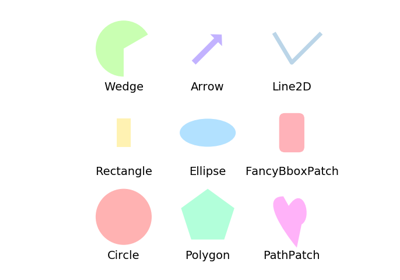Travis-CI:# matplotlib.patches.BoxStyle¶

class `matplotlib.patches.``BoxStyle`

`BoxStyle` is a container class which defines several boxstyle classes, which are used for `FancyBboxPatch`.

A style object can be created as:

```BoxStyle.Round(pad=0.2)
```

or:

```BoxStyle("Round", pad=0.2)
```

or:

```BoxStyle("Round, pad=0.2")
```

Following boxstyle classes are defined.

Class Name Attrs
Circle `circle` pad=0.3
DArrow `darrow` pad=0.3
LArrow `larrow` pad=0.3
RArrow `rarrow` pad=0.3
Round `round` pad=0.3,rounding_size=None
Round4 `round4` pad=0.3,rounding_size=None
Roundtooth `roundtooth` pad=0.3,tooth_size=None
Sawtooth `sawtooth` pad=0.3,tooth_size=None
Square `square` pad=0.3

An instance of any boxstyle class is an callable object, whose call signature is:

```__call__(self, x0, y0, width, height, mutation_size, aspect_ratio=1.)
```

and returns a `Path` instance. x0, y0, width and height specify the location and size of the box to be drawn. mutation_scale determines the overall size of the mutation (by which I mean the transformation of the rectangle to the fancy box). mutation_aspect determines the aspect-ratio of the mutation.

return the instance of the subclass with the given style name.

class `Circle`(pad=0.3)

A simple circle box.

Parameters: pad : float The amount of padding around the original box.
`transmute`(x0, y0, width, height, mutation_size)
class `DArrow`(pad=0.3)

(Double) Arrow Box

`transmute`(x0, y0, width, height, mutation_size)
class `LArrow`(pad=0.3)

(left) Arrow Box

`transmute`(x0, y0, width, height, mutation_size)
class `RArrow`(pad=0.3)

(right) Arrow Box

`transmute`(x0, y0, width, height, mutation_size)
class `Round`(pad=0.3, rounding_size=None)

A box with round corners.

rounding_size
`transmute`(x0, y0, width, height, mutation_size)
class `Round4`(pad=0.3, rounding_size=None)

Another box with round edges.

rounding_size
rounding size of edges. pad if None
`transmute`(x0, y0, width, height, mutation_size)
class `Roundtooth`(pad=0.3, tooth_size=None)

A rounded tooth box.

tooth_size
size of the sawtooth. pad* if None
`transmute`(x0, y0, width, height, mutation_size)
class `Sawtooth`(pad=0.3, tooth_size=None)

A sawtooth box.

tooth_size
size of the sawtooth. pad* if None
`transmute`(x0, y0, width, height, mutation_size)
class `Square`(pad=0.3)

A simple square box.

`transmute`(x0, y0, width, height, mutation_size)
## Examples using `matplotlib.patches.BoxStyle`¶